Examples

Chapter 11 Class 8 Direct and Inverse Proportions
Serial order wise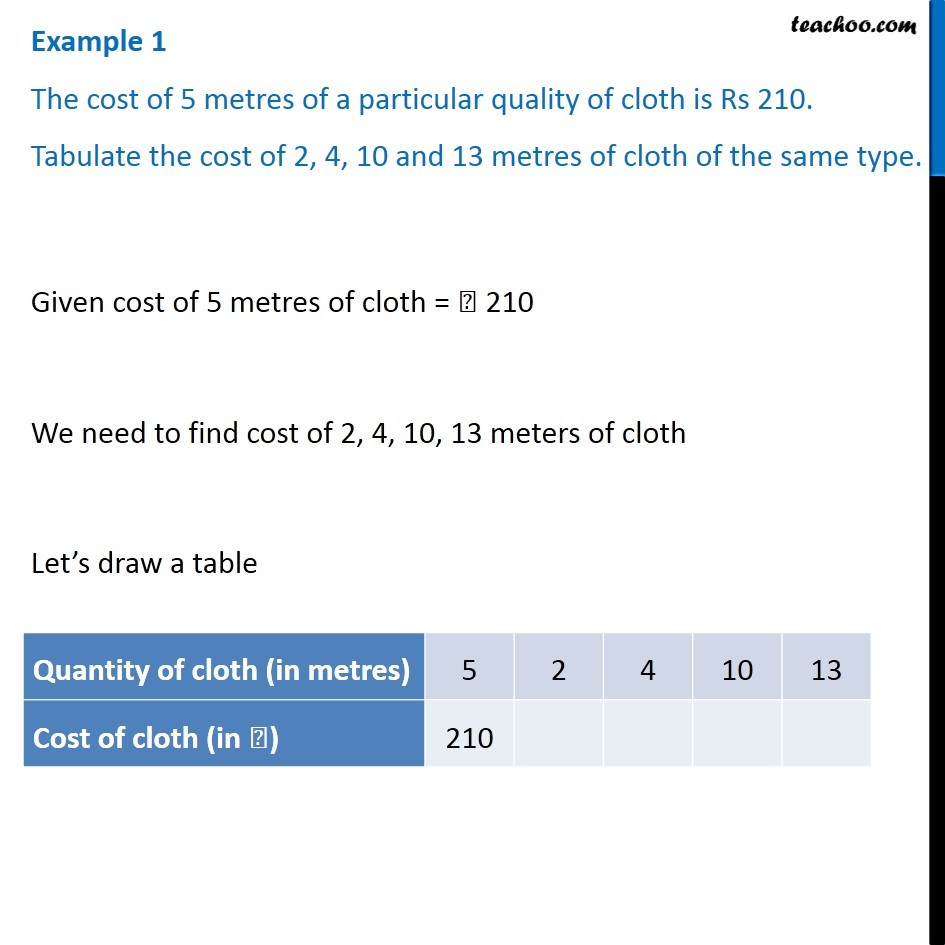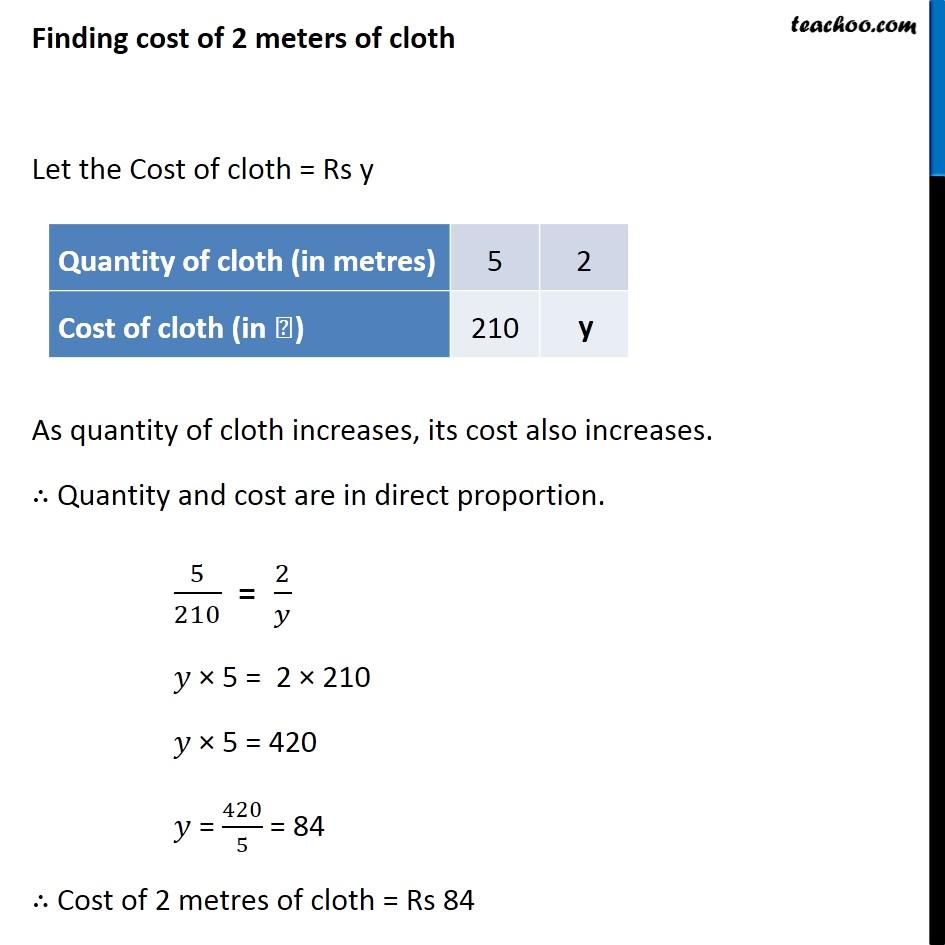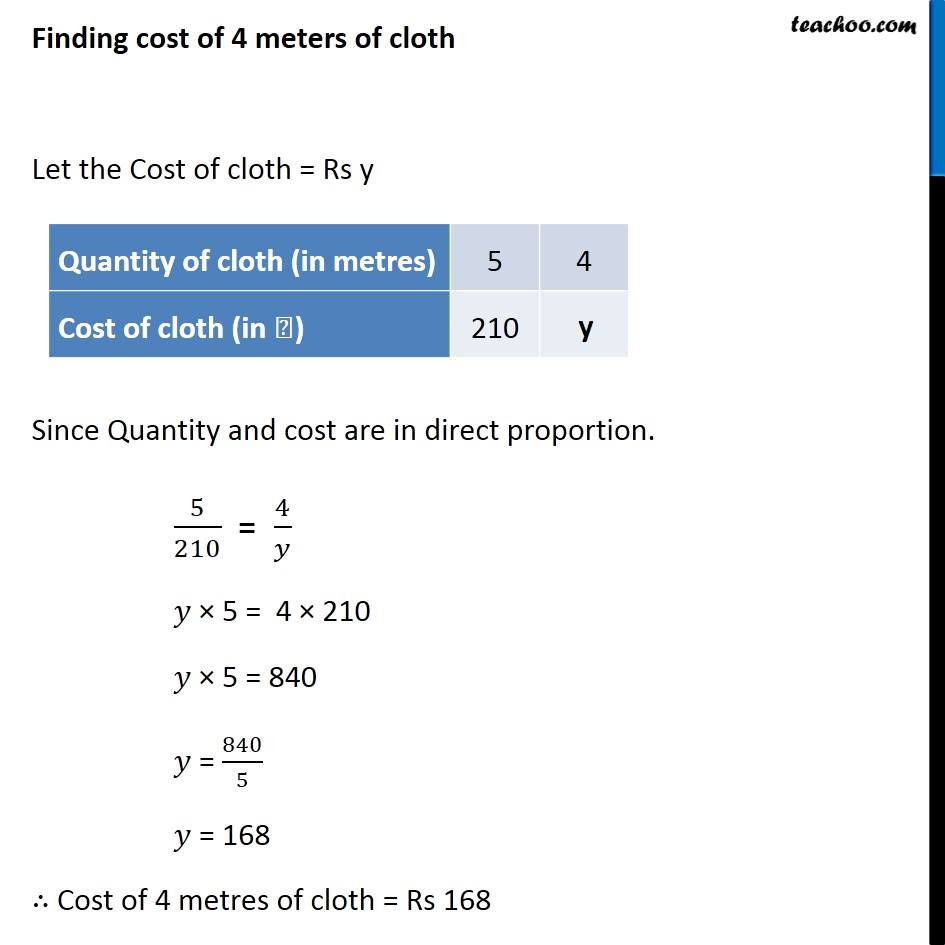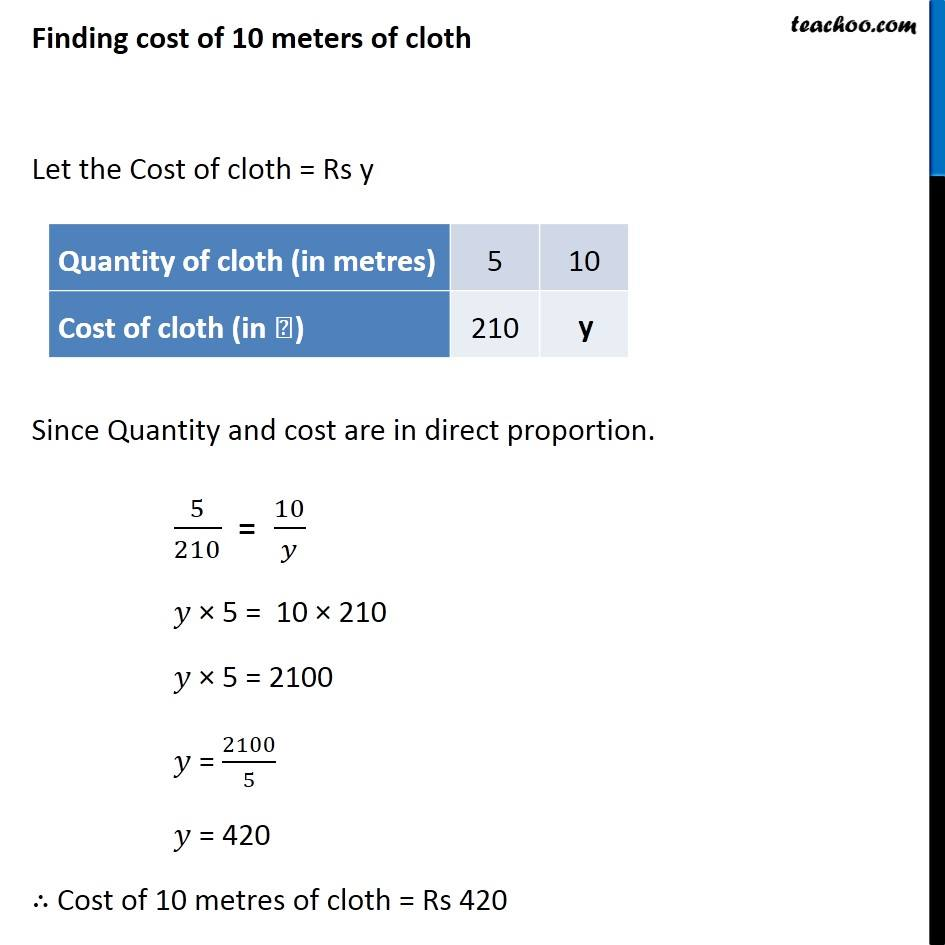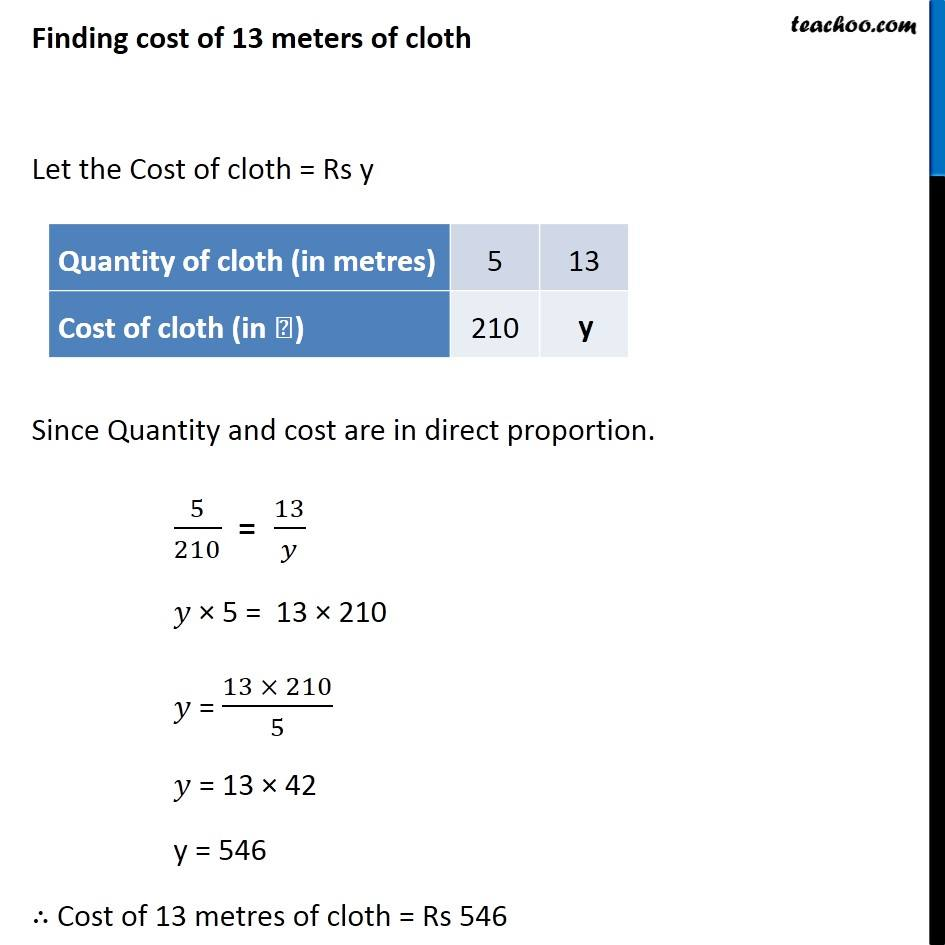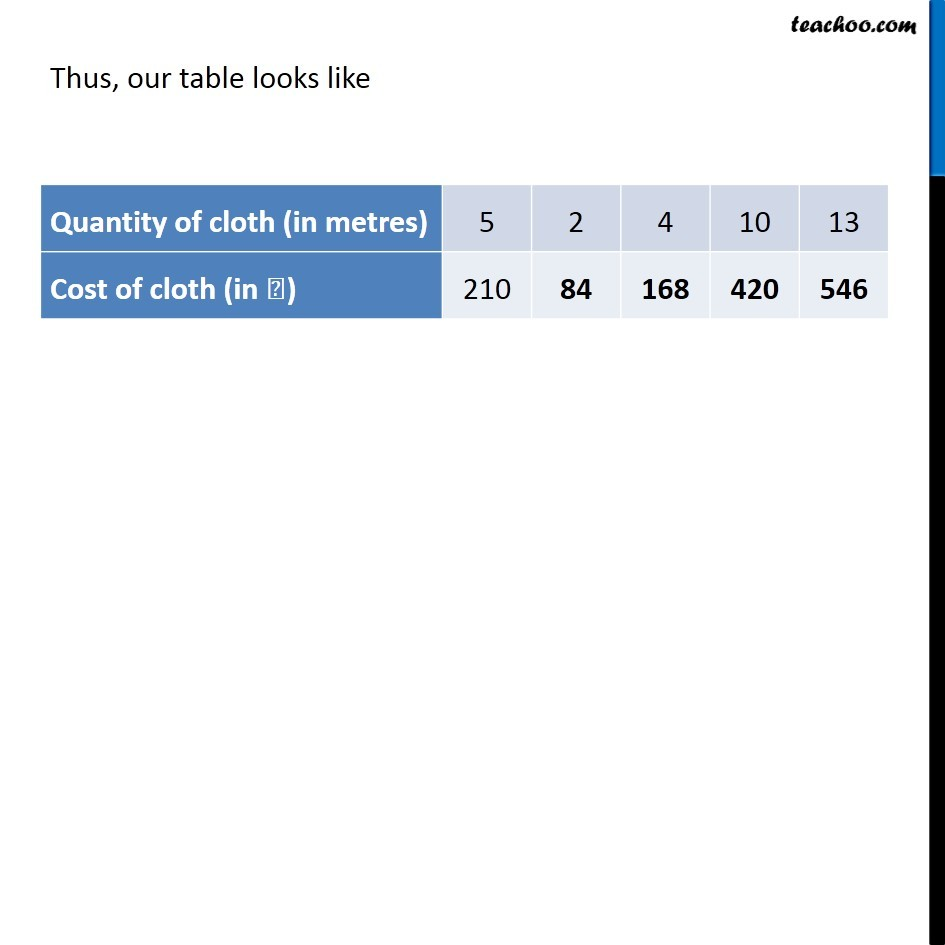Learn in your speed, with individual attention - Teachoo Maths 1-on-1 Class

### Transcript

Example 1 The cost of 5 metres of a particular quality of cloth is Rs 210. Tabulate the cost of 2, 4, 10 and 13 metres of cloth of the same type. Given cost of 5 metres of cloth = ₹ 210 We need to find cost of 2, 4, 10, 13 meters of cloth Let’s draw a table Finding cost of 2 meters of cloth Let the Cost of cloth = Rs y As quantity of cloth increases, its cost also increases. ∴ Quantity and cost are in direct proportion. 5/210 = 2/𝑦 𝑦 × 5 = 2 × 210 𝑦 × 5 = 420 𝑦 = 420/5 = 84 ∴ Cost of 2 metres of cloth = Rs 84 Finding cost of 4 meters of cloth Let the Cost of cloth = Rs y Since Quantity and cost are in direct proportion. 5/210 = 4/𝑦 𝑦 × 5 = 4 × 210 𝑦 × 5 = 840 𝑦 = 840/5 𝑦 = 168 ∴ Cost of 4 metres of cloth = Rs 168 Finding cost of 10 meters of cloth Let the Cost of cloth = Rs y Since Quantity and cost are in direct proportion. 5/210 = 10/𝑦 𝑦 × 5 = 10 × 210 𝑦 × 5 = 2100 𝑦 = 2100/5 𝑦 = 420 ∴ Cost of 10 metres of cloth = Rs 420 Finding cost of 13 meters of cloth Let the Cost of cloth = Rs y Since Quantity and cost are in direct proportion. 5/210 = 13/𝑦 𝑦 × 5 = 13 × 210 𝑦 = (13 × 210)/5 𝑦 = 13 × 42 y = 546 ∴ Cost of 13 metres of cloth = Rs 546 Thus, our table looks like